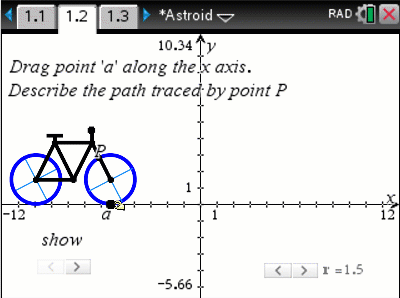# Activities

•• ##### AuthorAust Senior

60 Minutes

• ##### Device
•TI-Nspire™ CX CAS
• ##### Software

TI-Nspire™ CAS

4.5

## Astroid#### Activity Overview

Students start by considering the motion of a point on the wheel of a bicycle. With the aid of interactive diagrams in the TI-Nspire document, students go on to explore the case when the wheel rotating inside another resulting in a hypocycloid. Students generate the parametric form of the equation, use compound and double angle formulas to generate the equivalent Cartesian equations and use calculus to analyse features of the curves, including gradient and arc length.

#### Objectives

• Parametric form and graphs of a cycloids and epicycles
• Converting parametric equations to Cartesian
• Application of chain rule to related rates of change and implicit differentiation
• Derivatives of explicit and implicit functions
• Application of integration to arc lengths of curves
• Use of trigonometric identities including compound and double angle formulas

• Parameter
• Cartesian
• Implicit
• Cycloid
• Hypocycloid
• Locus